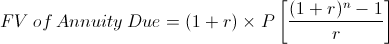# Annuity Due Payment - FV

Annuity Due Payment FV Calculator (Click Here or Scroll Down)The annuity due payment formula using future value is used to calculate each equal cash flow or payment of a series of cash flows when the future value is known. This formula is specific to annuities where the initial cash flow is received immediately.

Using the future value of an annuity due to calculate payments is required for different situations than when present value is used. For example, suppose that an individual would like to calculate the amount they would need to deposit per year, starting today, in an interest bearing account in order to have a certain balance after a certain period of time. The balance wanted after the allotted time would be considered the future value. The balance of the annuity would be increasing. This situation would require using the annuity due payment formula using future value.

However, if an individual would like to determine how much to withdraw from their current balance per year, starting today, in order for their current balance to last a certain period of time, then the balance would be decreasing. In this situation, the payment for the annuity due would be calculated from present value, or the initial balance.

## Example of the Annuity Due Payment Formula Using Future Value

As an example of the annuity due payment formula using future value, suppose that an individual would like to have \$5,000 saved within 5 years. The individual plans on making equal deposits per year starting today into an account that has an effective annual rate of 3%.

As with any other financial formula, it is important that the rate is expressed per period. For example, if the deposits are made monthly, then the monthly rate would be used. For this particular example, 3% is the effective annual rate and the deposits are made annually.

After putting the variables from this example into the annuity due payment formula using future value, the equation would beAfter solving, the amount to be deposited per year, starting today, would be \$914.34. Actual results may vary by a few cents due to rounding.

## How is the Annuity Due Payment Formula using Future Value Derived?

Determining the annuity due payment formula using future value first requires looking at the future value of annuity due formula, which isSince the payment is what is being calculated, both sides of this equation can be multiplied by the reciprocal of all variables on the right side, except for P, the payment. This results in the annuity due payment formula shown at the top of the page.

New to Finance?